# Reason + divisibility - math problems

#### Number of problems found: 106

• NumberWhat number should be placed instead of the asterisk in number 702*8 to get a number divisible by 6?
• Red and white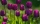Simona picked 63 tulips in the garden and tied bicolor bouquets for her girlfriends. The tulips were only red and white. She put as many tulips in each bouquet, three of which were always red. How much could Simon tear off white tulips? Write all the opti
• Twos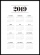Vojta started writing the number of this year 2019202020192020 into the workbook. .. And so he kept going. When he wrote 2020 digits, he no longer enjoyed it. How many twos did he write?
• Dance groupThe dance group formed groups of 4, 5, and 6 members. Always one dancer remains. How many dancers were there in the whole group?
• Two cars on ring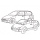There were two cars on the round track (ring) in the adjacent tracks, the first car in the inner track, the second in the outer track. Both cars started at the same time from one starting track. The first toy car drove every four laps simultaneously as th
• Three numbersWe have three different non-zero digits. We will create all 3 digits numbers from them to use all 3 figures in each number. We add all the created numbers, and we get the sum of 1554. What were the numbers?
• Hens and pigs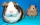Hens and pigs have 46 feet in total. At least how much can heads have?
• Reminder and quotientThere are given the number C = 281, D = 201. Find the highest natural number S so that the C:S, D:S are with the remainder of 1,
• The HotelThe Holiday Hotel has the same number of rooms on each floor. Rooms are numbered with natural numerals sequentially from the first floor, no number is omitted, and each room has a different number. Three tourists arrived at the hotel. The first one was in
• Six-digit primesFind all six-digit prime numbers that contain each one of digits 1,2,4,5,7, and 8 just once. How many are they?
• The florist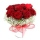The florist had 200 roses in the morning. During the day, more than half sold it. From the remaining roses, it will tie the bouquet. If a bouquet of 3, 4, 5, or 6 roses are bound, one always remains. How many roses from the morning shipment sold?
• Chocolate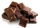I have a box of chocolate - white, milk and dark. The ratio of white to milk with dark is 3: 4. The ratio of white and milk to dark is 17: 4. Calculate what is the ratio between white, milk, dark chocolate.
• Four poplarsFour poplars are growing along the way. The distances between them are 35 m, 14 m, and 91 m. At least how many poplars need to be dropped to create the same spacing between the trees? How many meters will it be?
• Bricks pyramidHow many 50cm x 32cm x 30cm brick needed to built a 272m x 272m x 278m pyramid?
• Odd/even numberPick any number. If that number is even, divide it by 2. If it's odd, multiply it by three and add one. Now repeat the process with your new number. If you keep going, you'll eventually end up at one every time. Prove.
• Year 2018The product of the three positive numbers is 2018. What are the numbers?
• Trees in alleyThere are four trees in the alley between which the distances are 35m, 15m and 95m. Trees must be laid in the spaces so that the distance is the same and the maximum. How many trees will they put in and what will be the distance between them?
• MO C–I–1 2018An unknown number is divisible by just four numbers from the set {6, 15, 20, 21, 70}. Determine which ones.
• Clock's gears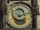In the clock machine, three gears fit together. The largest has 168 teeth, the middle 90 teeth, and the smallest 48 teeth. The middle wheel turns around its axis in 90 seconds. How many times during the day do all the gears meet in the starting position?
• Inverted nineIn the hotel Inverted Nine, each hotel room number is divisible by 6. How many rooms can we count with the three-digit number registered by digits 1,8,7,4,9?

Do you have an exciting math question or word problem that you can't solve? Ask a question or post a math problem, and we can try to solve it.

We will send a solution to your e-mail address. Solved examples are also published here. Please enter the e-mail correctly and check whether you don't have a full mailbox.

Reason - math problems. Divisibility - math problems.